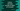# How to calculate the average sum of all digits of a number in python## How to calculate the average sum of all digits of a number in python:

In this post, we will learn how to calculate the average sum of all digits of a number. The average sum is calculated by dividing the sum of all digits of a number by total digits in that number.

For example, if we want to calculate it for the number 1234, it will be 2.5.

The sum of all digits of 1234 is 1 + 2 + 3 + 4 = 10. It has 4 digits. So, the average value is 10/4 = 2.5.

### Algorithm to calculate the average sum of all digits of a number:

We can use the below algorithm to calculate the average sum of all digits of a number:

• Take the number as input from the user.
• Use one loop to run until the value of the number is 0:

• Pick the last digit of the number and add it to a sum variable. This variable is initialized as 0. Also, increment the value of a counter to calculate the total digits.
• Remove the last digit of the number by divide it by 10.
• Run this loop until the number is 0.
• Once the loop will end, the sum variable will hold the sum of all digits of the number and counter variable will hold the total digits of that number. Divide sum variable by counter to find the average.

## Python program:

Below is the complete program that calculates the average sum of all digits of a number:

``````def find_avg_sum(num):
count = 0
sum = 0
while(num > 0):
sum += num % 10
count += 1
num = int(num/10)
return sum/count

given_number = int(input('Enter a number: '))

print('Average sum of all digits : {}'.format(find_avg_sum(given_number)))``````

Here,

• It takes one number as input from the user and assigns that value to the variable given_number.
• findavgsum method is used to find the average sum of all digits of a number. It takes one number as its argument and returns the average sum of all digits.

• count and sum are variables to store the digit count and sum of all digits for a number.
• The while loop runs till the value of num is greater than 0.
• num % 10 gives the last digit of a number. We are adding it to sum variable. We are also incrementing the value of count by 1 as we need to count the number of digits as well.
• Dividing the number by 10 and converting this value to int will remove the last digit of a number.
• This while loop will stop once the value of num become 0.
• It returns sum/count. sum variable holds the sum of all digits of the number and count holds the total number of digits in the number. So, sum/count is the required average value.

### Sample output:

If you run this program, it will give output as like below:

``````Enter a number: 1234
Average sum of all digits : 2.5

Enter a number: 12345
Average sum of all digits : 3.0``````

As you can see here, it calculates the average sum of all digits of the numbers.

### Conclusion:

In this post, we learned how to calculate the average sum of all digits of a number in Python. We have learned how to iterate through the digits of a number, how to use a separate function, how to use a while loop and how to do basic arithmatic in python with this example. You can try to find the average of all digits at even position and at odd position for a number.